# ORA-01476: divisor is equal to zero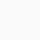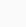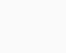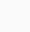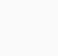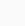•ANKUSH THAVALI
• 11 Nov, 2021

## ORA-01476: divisor is equal to zero#### ORA-01476 is a very general error, and it comes when we try to divide any number by 0. In mathematics, DIVIDEND/0 has no meaning. Or we can simply say division by zero is undefined. In Oracle database when we try to divide by 0, Oracle Database throws an exception - "ORA-01476: divisor is equal to zero". For Example:

ankush@thavali> WITH T AS ( 2 SELECT 1 DIVIDEND, 0 DIVISOR FROM DUAL 3 ) 4 SELECT DIVIDEND/DIVISOR AS QUOTIENT FROM T; SELECT DIVIDEND/DIVISOR AS QUOTIENT FROM T * ERROR at line 4: ORA-01476: divisor is equal to zero

### 1. Case When Then

#### Here we simply return NULL is DIVISOR is ZERO, otherwise we divide.

```ankush@thavali> WITH T AS (
2   SELECT 1 DIVIDEND, 0 DIVISOR FROM DUAL
3  )
4  SELECT
5     CASE WHEN DIVISOR = 0
6     THEN NULL
7     ELSE DIVIDEND/DIVISOR
8     END QUOTIENT
9  FROM T;

QUOTIENT
----------
```

### 2. NULLIF(expr1, expr2)

#### NULLIF compares expr1 and expr2. If they are equal, then the function returns NULL. If they are not equal, then the function returns EXPR1. In Oracle Database any mathematical operation involving NULL is evaluated to NULL - DIVIDEND/NULL = NULL

```ankush@thavali&gt; WITH T AS (
2 SELECT 1 DIVIDEND, 0 DIVISOR FROM DUAL
3 )
4 SELECT
5 DIVIDEND/NULLIF(DIVISOR ,0) QUOTIENT
6 FROM T;

QUOTIENT
----------
```